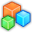# Microsoft Small BasicProgram Listing: PWG193
'This was made from the example "Paint Prgram"
'You can use custom colors
'Just press the first letter of the color
'There are 7 colors

GraphicsWindow.BackgroundColor = "Black"
GraphicsWindow.PenColor = "White"
GraphicsWindow.MouseDown = OnMouseDown
GraphicsWindow.MouseMove = OnMouseMove
GraphicsWindow.KeyDown = OnKeyDown

Sub OnKeyDown
If (GraphicsWindow.LastKey = "R") Then
GraphicsWindow.PenColor = "Red"
EndIf
If (GraphicsWindow.LastKey = "W") Then
GraphicsWindow.PenColor = "White"
endif
If (GraphicsWindow.LastKey = "B") Then
GraphicsWindow.PenColor = "Blue"
endif
If (GraphicsWindow.LastKey = "G") Then
GraphicsWindow.PenColor = "Green"
endif
If (GraphicsWindow.LastKey = "P") Then
GraphicsWindow.PenColor = "Purple"
endif
If (GraphicsWindow.LastKey = "Y") Then
GraphicsWindow.PenColor = "Yellow"
endif
If (GraphicsWindow.LastKey = "O") Then
GraphicsWindow.PenColor = "Orange"
endif
If (GraphicsWindow.LastKey = "R") Then
GraphicsWindow.PenColor = "Red"
EndIf
If (GraphicsWindow.LastKey = "W") Then
GraphicsWindow.PenColor = "White"
endif
If (GraphicsWindow.LastKey = "B") Then
GraphicsWindow.PenColor = "Blue"
endif
If (GraphicsWindow.LastKey = "G") Then
GraphicsWindow.PenColor = "Green"
endif
If (GraphicsWindow.LastKey = "P") Then
GraphicsWindow.PenColor = "Purple"
endif
If (GraphicsWindow.LastKey = "Y") Then
GraphicsWindow.PenColor = "Yellow"
endif
If (GraphicsWindow.LastKey = "O") Then
GraphicsWindow.PenColor = "Orange"
endif
EndSub

Sub OnMouseDown
prevX = GraphicsWindow.MouseX
prevY = GraphicsWindow.MouseY
EndSub

Sub OnMouseMove
x = GraphicsWindow.MouseX
y = GraphicsWindow.MouseY
If (Mouse.IsLeftButtonDown) then
GraphicsWindow.DrawLine(prevX, prevY, x, y)
endif
prevX = x
prevY = y
EndSub